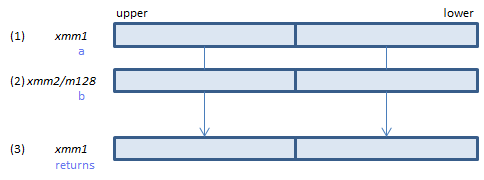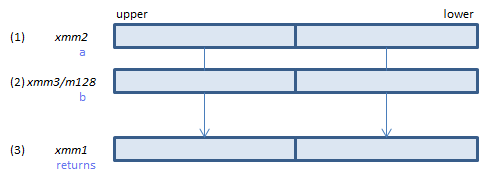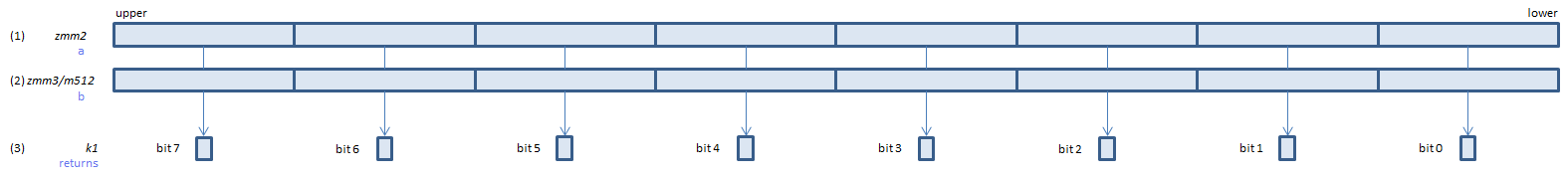﻿ cmpordpd

## CMPORDPD - CoMPare ORDered Packed Double

CMPORDPD xmm1, xmm2/m128    (S2
__m128d _mm_cmpord_pd(__m128d a, __m128d b)For each double, if neither of (1)(2) is NaN, set 1, else set 0, to all bits of the corresponding double of (3).
VCMPORDPD xmm1, xmm2, xmm3/m128    (V1
__m128d _mm_cmpord_pd(__m128d a, __m128d b)For each double, if neither of (1)(2) is NaN, set 1, else set 0, to all bits of the corresponding double of (3).
VCMPORDPD ymm1, ymm2, ymm3/m256    (V1
__m256d _mm256_cmp_pd(__m256d a, __m256d b, _CMP_ORD_Q)For each double, if neither of (1)(2) is NaN, set 1, else set 0, to all bits of the corresponding double of (3).
VCMPORDPD k1{k2}, xmm2, xmm3/m128/m64bcst    (V5+VLFor each double, if neither of (1)(2) is NaN, set 1, else set 0, to the corresponding bit of (3).
If k2 bit is 0, the comparison is not done and the corresponding bit of (3) is set to zero. Upper bits of (3) are zero cleared.
VCMPORDPD k1{k2}, ymm2, ymm3/m256/m64bcst    (V5+VLFor each double, if neither of (1)(2) is NaN, set 1, else set 0, to the corresponding bit of (3).
If k2 bit is 0, the comparison is not done and the corresponding bit of (3) is set to zero. Upper bits of (3) are zero cleared.
VCMPORDPD k1{k2}, zmm2, zmm3/m512/m64bcst{sae}    (V5